# Selina Solutions Concise Maths Class 7 Chapter 9: Profit, Loss and Discount Exercise 9A

Selina Solutions Concise Maths Class 7 Chapter 9 Profit, Loss and Discount Exercise 9A provides students with a clear cut picture of the basic concepts, which are important for the exam. The steps to be followed in determining the selling price is the important concept covered under this exercise. Students are advised to practise the exercise wise problems to score well in the Class 7 exam. In order to gain a hold on the concepts, Selina Solutions Concise Maths Class 7 Chapter 9 Profit, Loss and Discount Exercise 9A, free PDF can be downloaded from the links which are available here.

## Selina Solutions Concise Maths Class 7 Chapter 9: Profit, Loss and Discount Exercise 9A Download PDF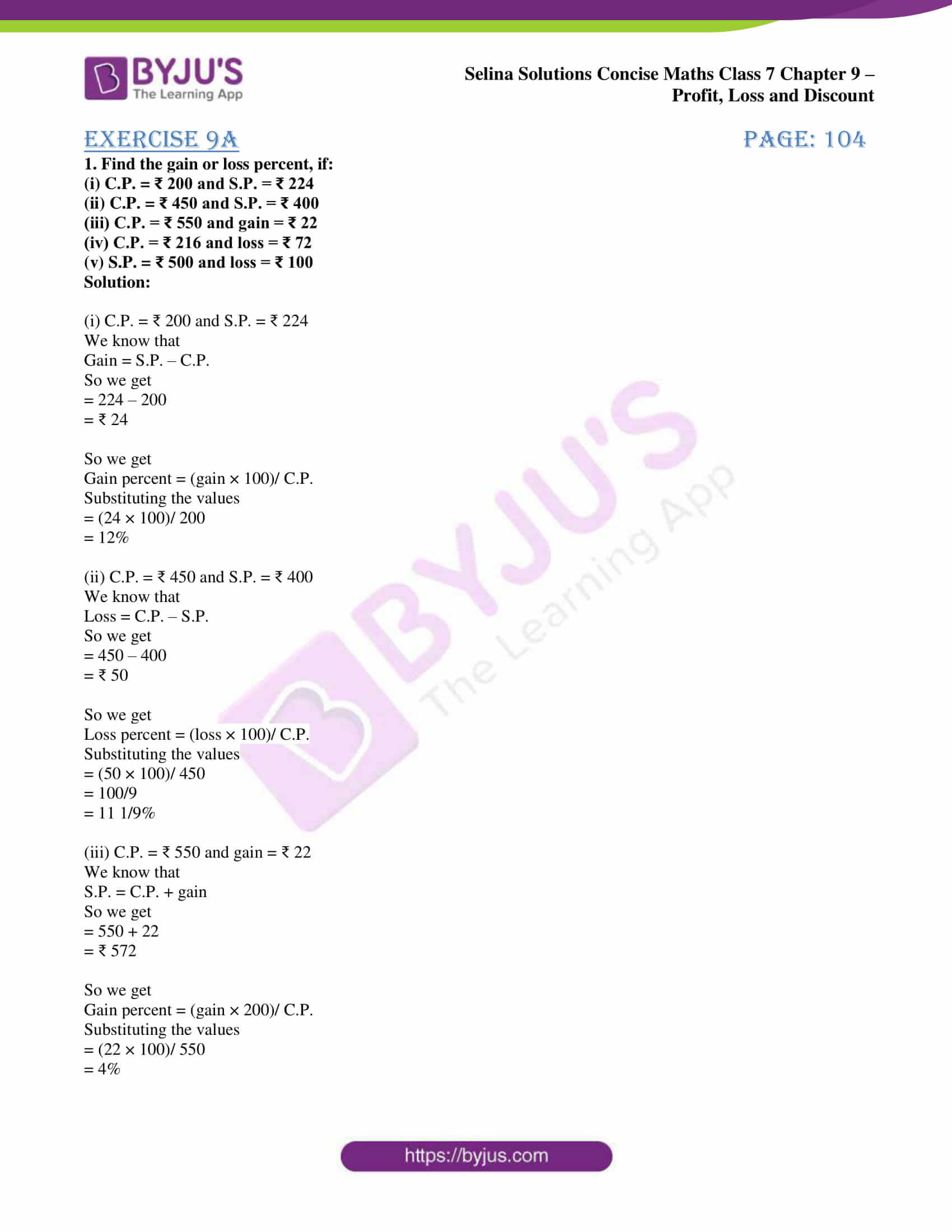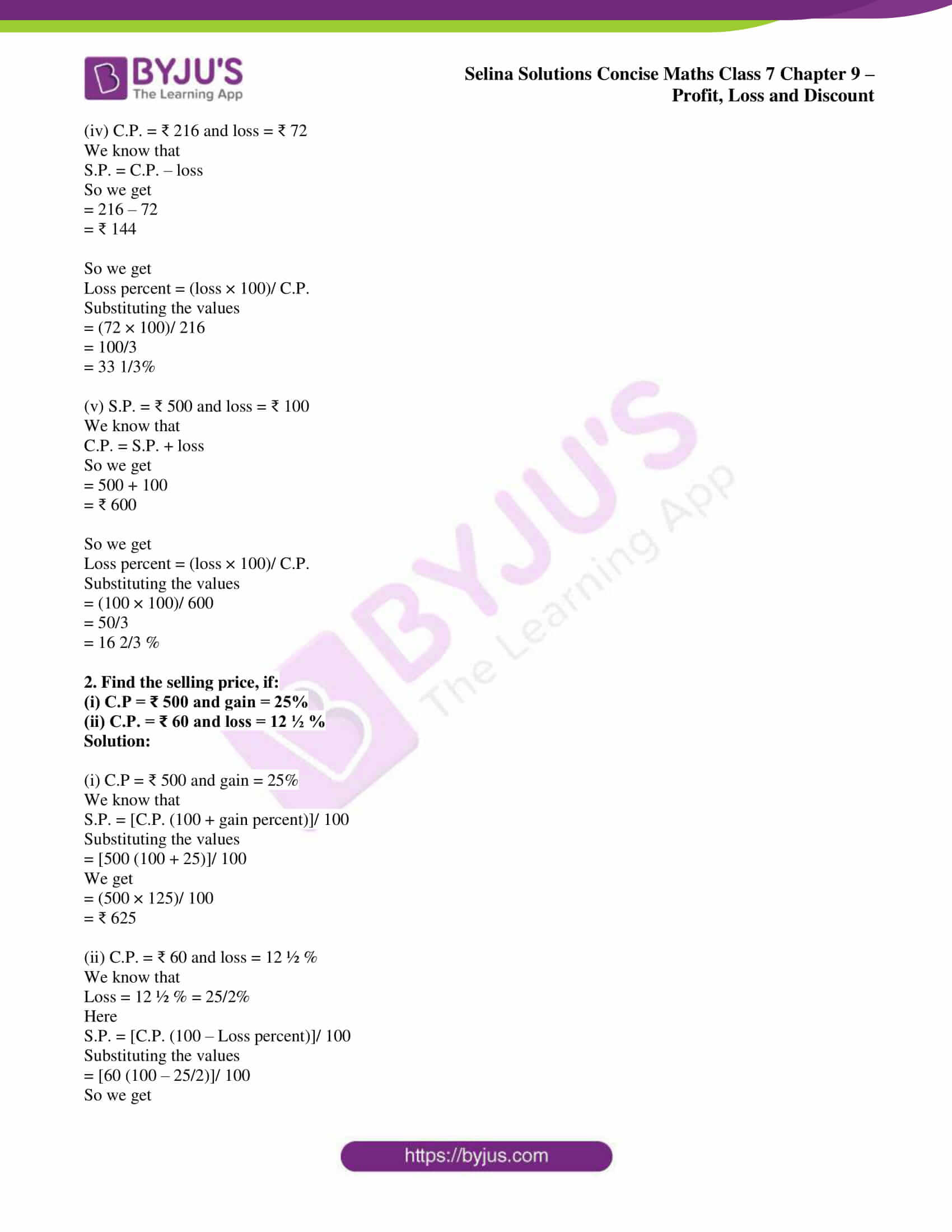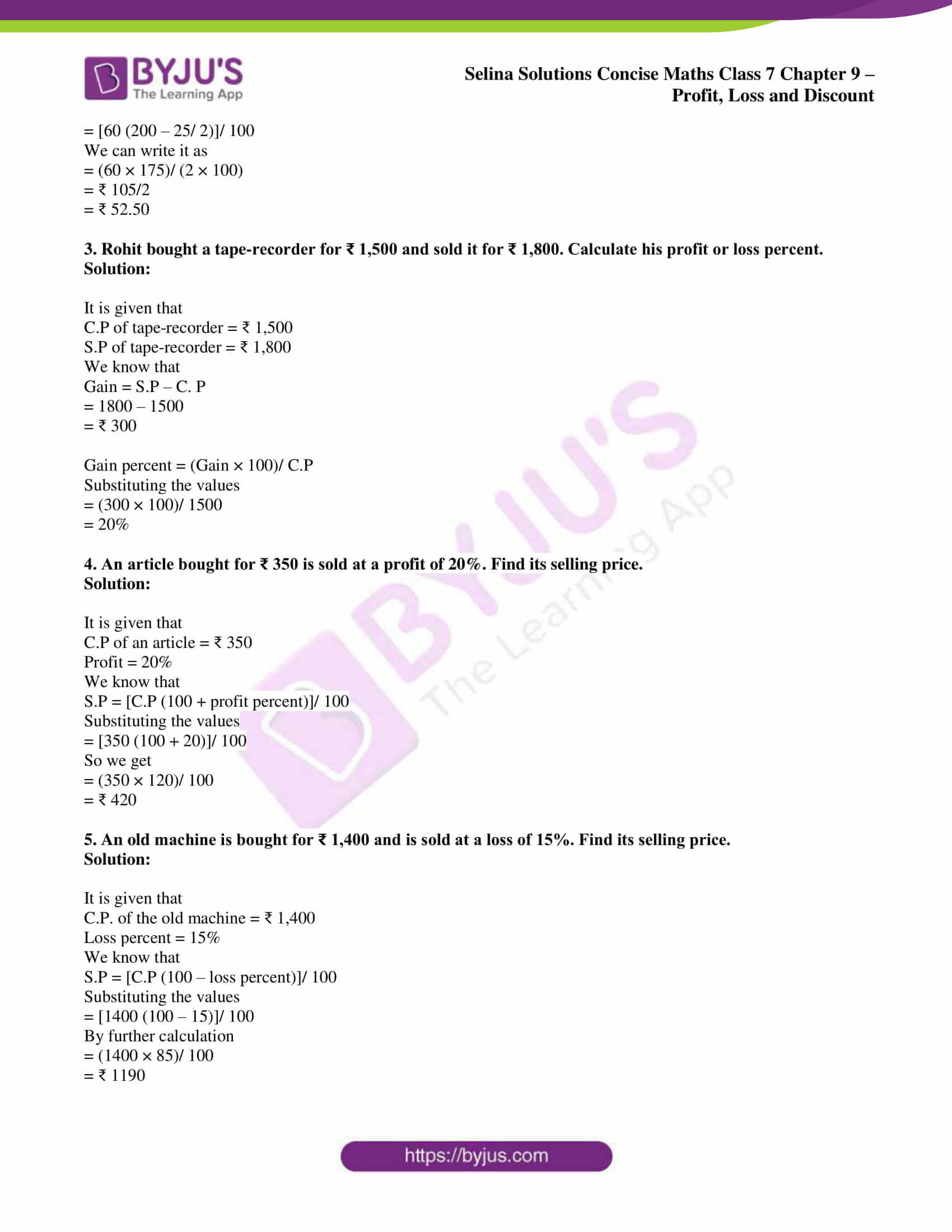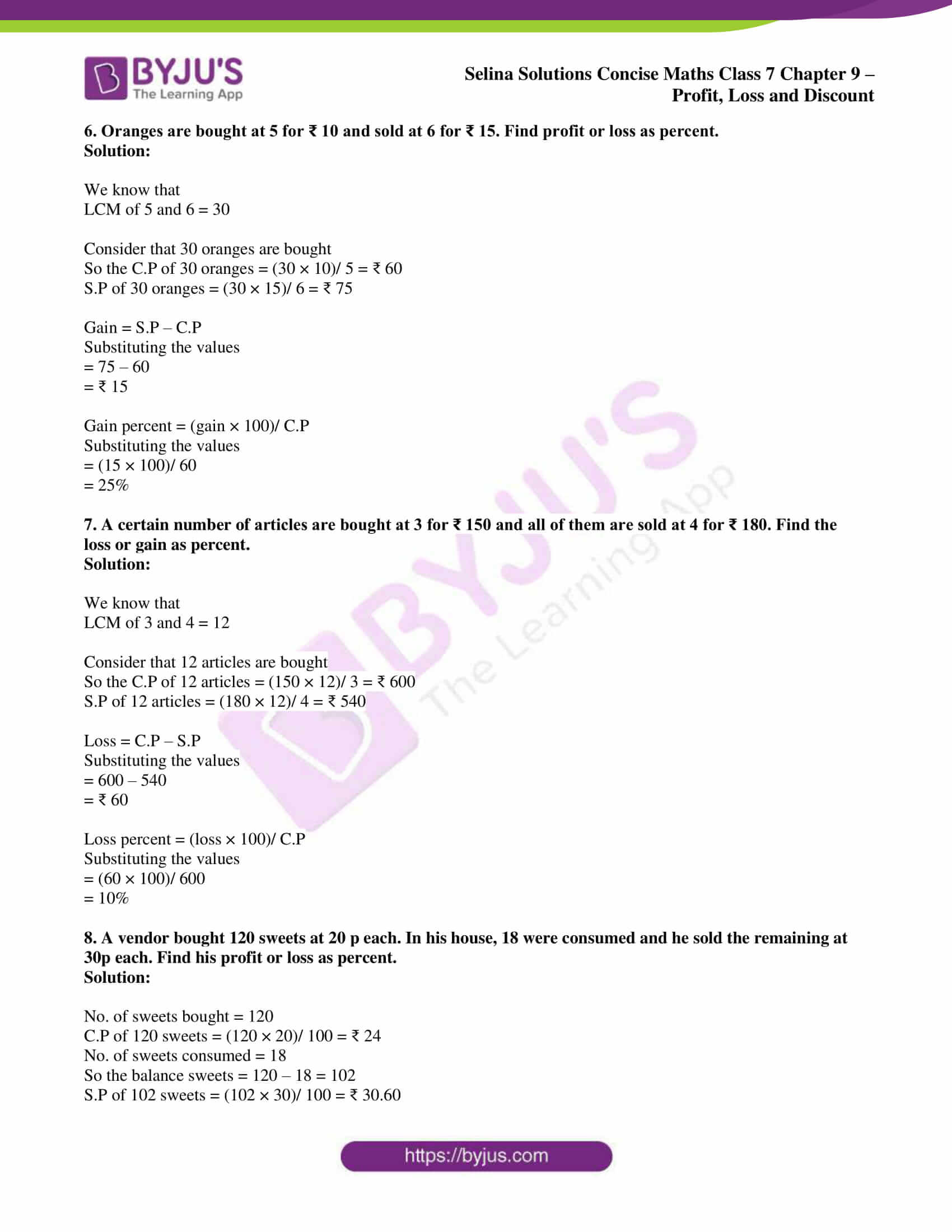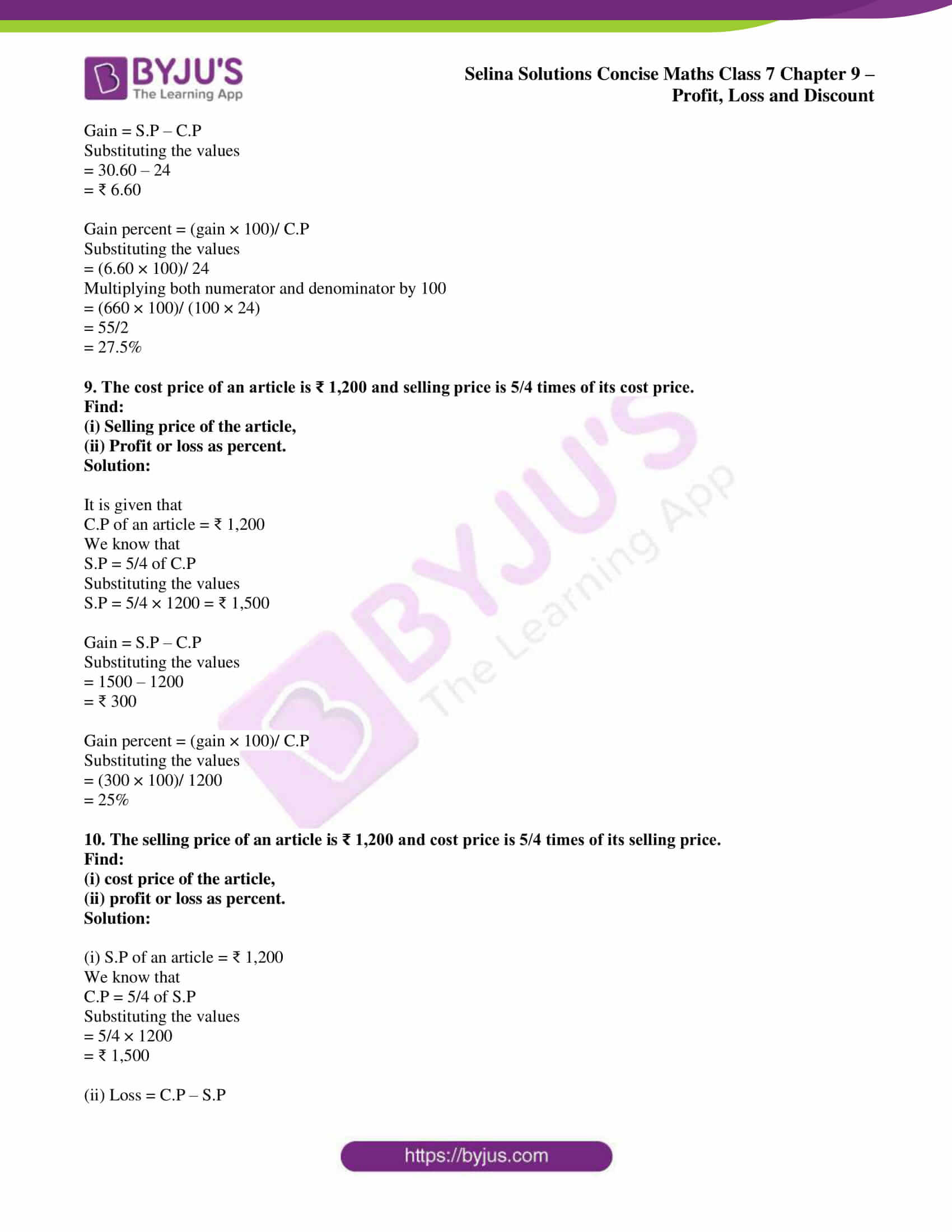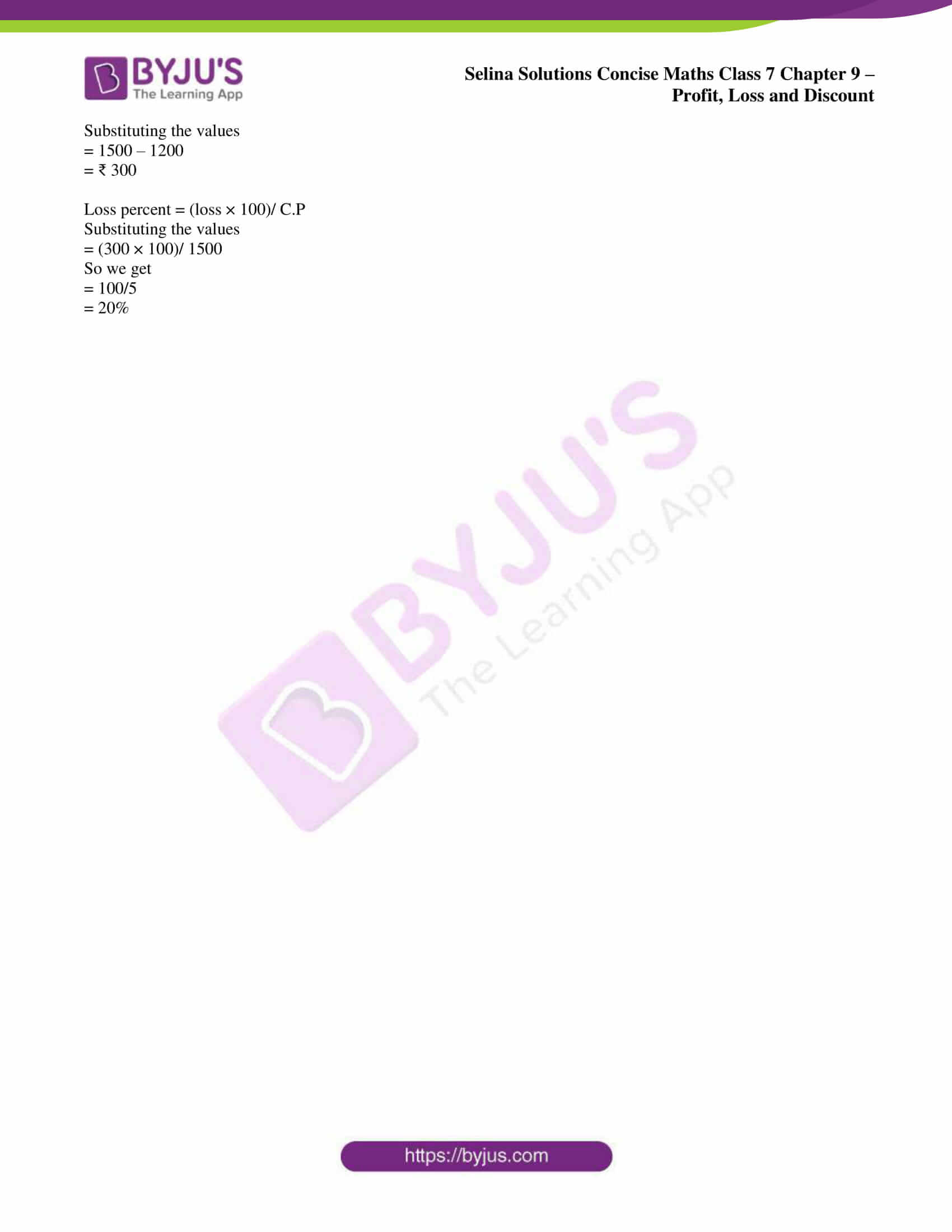### Access Selina Solutions Concise Maths Class 7 Chapter 9: Profit, Loss and Discount Exercise 9A

#### Exercise 9A page: 104

1. Find the gain or loss percent, if:

(i) C.P. = ₹ 200 and S.P. = ₹ 224

(ii) C.P. = ₹ 450 and S.P. = ₹ 400

(iii) C.P. = ₹ 550 and gain = ₹ 22

(iv) C.P. = ₹ 216 and loss = ₹ 72

(v) S.P. = ₹ 500 and loss = ₹ 100

Solution:

(i) C.P. = ₹ 200 and S.P. = ₹ 224

We know that

Gain = S.P. – C.P.

So we get

= 224 – 200

= ₹ 24

So we get

Gain percent = (gain × 100)/ C.P.

Substituting the values

= (24 × 100)/ 200

= 12%

(ii) C.P. = ₹ 450 and S.P. = ₹ 400

We know that

Loss = C.P. – S.P.

So we get

= 450 – 400

= ₹ 50

So we get

Loss percent = (loss × 100)/ C.P.

Substituting the values

= (50 × 100)/ 450

= 100/9

= 11 1/9%

(iii) C.P. = ₹ 550 and gain = ₹ 22

We know that

S.P. = C.P. + gain

So we get

= 550 + 22

= ₹ 572

So we get

Gain percent = (gain × 200)/ C.P.

Substituting the values

= (22 × 100)/ 550

= 4%

(iv) C.P. = ₹ 216 and loss = ₹ 72

We know that

S.P. = C.P. – loss

So we get

= 216 – 72

= ₹ 144

So we get

Loss percent = (loss × 100)/ C.P.

Substituting the values

= (72 × 100)/ 216

= 100/3

= 33 1/3%

(v) S.P. = ₹ 500 and loss = ₹ 100

We know that

C.P. = S.P. + loss

So we get

= 500 + 100

= ₹ 600

So we get

Loss percent = (loss × 100)/ C.P.

Substituting the values

= (100 × 100)/ 600

= 50/3

= 16 2/3 %

2. Find the selling price, if:

(i) C.P = ₹ 500 and gain = 25%

(ii) C.P. = ₹ 60 and loss = 12 ½ %

Solution:

(i) C.P = ₹ 500 and gain = 25%

We know that

S.P. = [C.P. (100 + gain percent)]/ 100

Substituting the values

= [500 (100 + 25)]/ 100

We get

= (500 × 125)/ 100

= ₹ 625

(ii) C.P. = ₹ 60 and loss = 12 ½ %

We know that

Loss = 12 ½ % = 25/2%

Here

S.P. = [C.P. (100 – Loss percent)]/ 100

Substituting the values

= [60 (100 – 25/2)]/ 100

So we get

= [60 (200 – 25/ 2)]/ 100

We can write it as

= (60 × 175)/ (2 × 100)

= ₹ 105/2

= ₹ 52.50

3. Rohit bought a tape-recorder for ₹ 1,500 and sold it for ₹ 1,800. Calculate his profit or loss percent.

Solution:

It is given that

C.P of tape-recorder = ₹ 1,500

S.P of tape-recorder = ₹ 1,800

We know that

Gain = S.P – C. P

= 1800 – 1500

= ₹ 300

Gain percent = (Gain × 100)/ C.P

Substituting the values

= (300 × 100)/ 1500

= 20%

4. An article bought for ₹ 350 is sold at a profit of 20%. Find its selling price.

Solution:

It is given that

C.P of an article = ₹ 350

Profit = 20%

We know that

S.P = [C.P (100 + profit percent)]/ 100

Substituting the values

= [350 (100 + 20)]/ 100

So we get

= (350 × 120)/ 100

= ₹ 420

5. An old machine is bought for ₹ 1,400 and is sold at a loss of 15%. Find its selling price.

Solution:

It is given that

C.P. of the old machine = ₹ 1,400

Loss percent = 15%

We know that

S.P = [C.P (100 – loss percent)]/ 100

Substituting the values

= [1400 (100 – 15)]/ 100

By further calculation

= (1400 × 85)/ 100

= ₹ 1190

6. Oranges are bought at 5 for ₹ 10 and sold at 6 for ₹ 15. Find profit or loss as percent.

Solution:

We know that

LCM of 5 and 6 = 30

Consider that 30 oranges are bought

So the C.P of 30 oranges = (30 × 10)/ 5 = ₹ 60

S.P of 30 oranges = (30 × 15)/ 6 = ₹ 75

Gain = S.P – C.P

Substituting the values

= 75 – 60

= ₹ 15

Gain percent = (gain × 100)/ C.P

Substituting the values

= (15 × 100)/ 60

= 25%

7. A certain number of articles are bought at 3 for ₹ 150 and all of them are sold at 4 for ₹ 180. Find the loss or gain as percent.

Solution:

We know that

LCM of 3 and 4 = 12

Consider that 12 articles are bought

So the C.P of 12 articles = (150 × 12)/ 3 = ₹ 600

S.P of 12 articles = (180 × 12)/ 4 = ₹ 540

Loss = C.P – S.P

Substituting the values

= 600 – 540

= ₹ 60

Loss percent = (loss × 100)/ C.P

Substituting the values

= (60 × 100)/ 600

= 10%

8. A vendor bought 120 sweets at 20 p each. In his house, 18 were consumed and he sold the remaining at 30p each. Find his profit or loss as percent.

Solution:

No. of sweets bought = 120

C.P of 120 sweets = (120 × 20)/ 100 = ₹ 24

No. of sweets consumed = 18

So the balance sweets = 120 – 18 = 102

S.P of 102 sweets = (102 × 30)/ 100 = ₹ 30.60

Gain = S.P – C.P

Substituting the values

= 30.60 – 24

= ₹ 6.60

Gain percent = (gain × 100)/ C.P

Substituting the values

= (6.60 × 100)/ 24

Multiplying both numerator and denominator by 100

= (660 × 100)/ (100 × 24)

= 55/2

= 27.5%

9. The cost price of an article is ₹ 1,200 and selling price is 5/4 times of its cost price.

Find:

(i) Selling price of the article,

(ii) Profit or loss as percent.

Solution:

It is given that

C.P of an article = ₹ 1,200

We know that

S.P = 5/4 of C.P

Substituting the values

S.P = 5/4 × 1200 = ₹ 1,500

Gain = S.P – C.P

Substituting the values

= 1500 – 1200

= ₹ 300

Gain percent = (gain × 100)/ C.P

Substituting the values

= (300 × 100)/ 1200

= 25%

10. The selling price of an article is ₹ 1,200 and cost price is 5/4 times of its selling price.

Find:

(i) cost price of the article,

(ii) profit or loss as percent.

Solution:

(i) S.P of an article = ₹ 1,200

We know that

C.P = 5/4 of S.P

Substituting the values

= 5/4 × 1200

= ₹ 1,500

(ii) Loss = C.P – S.P

Substituting the values

= 1500 – 1200

= ₹ 300

Loss percent = (loss × 100)/ C.P

Substituting the values

= (300 × 100)/ 1500

So we get

= 100/5

= 20%